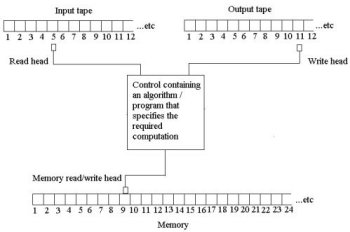A# Turing machineA Turing machine is an abstract model of computer execution and storage introduced in 1936 by Alan Turing to give a mathematically precise definition of algorithm. A Turing machine can be thought of as a black box that carries out a calculation of some kind on an input number. If the calculation reaches a conclusion, or halts, then an output number is returned. Otherwise, the machine theoretically carries on forever. There are an infinite number of Turing machines, as there are an infinite number of calculations that can be done with a finite list of rules. A Turing machine that can simulate any other Turing machine is called a universal Turing machine. The concept of Turing machines is still widely used in theoretical computer science, especially in complexity theory and the theory of computation.